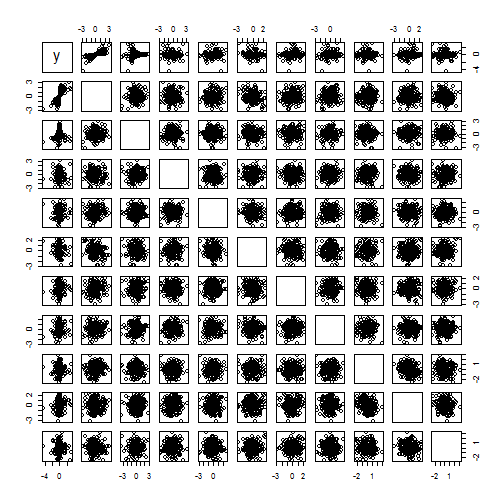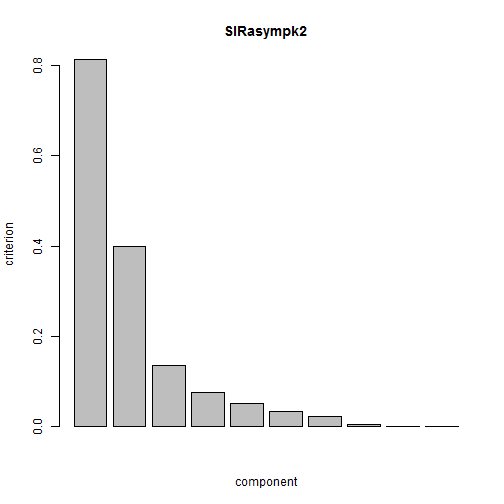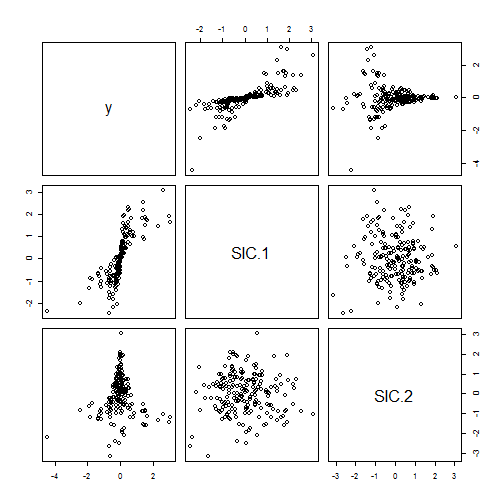## Sliced inverse regression

Denote the $$p$$-variate predictors $$x_i$$, $$i=1,…,n$$ with corresponding responses $$y_i$$. The predictors are assumed to follow the model $x_i = A s_i + b,$ where $$A$$ is a non-singular $$p \times p$$ matrix, $$b$$ a $$p$$-vector and the random vector $$s$$ can be decomposed into $$(s_1^T,s_2^T)^T$$ with $$E(s)=0$$ and $$Cov(s)=I_p$$ where $$s_1$$ has dimension $$k$$ and $$s_2$$ dimension $$p-k$$. It is then assumed that $$s_1$$ is the signal part, the interesting components, which are relevant to model $$y$$, whereas $$s_2$$ is the noise part.

The working model assumption is then that $(y,s_1^T)^T \ is \ independent \ of \ s_2.$ Defining $$S_1=E((x-b)(x-b)^T)$$ and $$S_2=E(E(x-b|y)E(x-b|y)^T)$$ the sliced inverse regression (SIR) can be interpreted as finding the transformation matrix $$W$$ such that $W S_1 W^T = I_p \ and \ W S_2 W^T = D,$ where $$D$$ is a diagonal matrix with diagonal elements $$d_1 \geq d_2 \geq … \geq d_k > d_{k+1} = … = d_p = 0$$.

In practice $$S_2$$ is estimated by approximating $$E(x-b|y)$$ by dividing $$y$$ into $$h$$ slices where in this package $$y$$ is divided into $$h$$ intervals containing an equal number of observations.

The practical problem is to decide then the value $$k$$.

### Asymptotic test a specific value of $$k$$

For an asymptotic test using the test statistic $T= n\sum_{i=k+1}^p d_i,$ the limiting distribution under the null is then $$T \sim \chi^2_{(p-k)(h-k-1)}$$. Therefore for the hypothetical value $$k$$ and the number of slices $$h$$ is required that $$k \geq h-1$$.

### Bootstrapping test a specific value of $$k$$

Bootstrap tests can be constructed as follows

1. Compute $$\hat s_i = \hat W(x_i - \bar x_i)$$, and decompose into $$\hat s_{i,1}$$ and $$\hat s_{i,2}$$.
2. Sample a bootstrap sample $$(y_i^*, \hat s_{i,1}^*)$$ of size $$n$$ from $$(y_i, \hat s_{i,1})$$.
3. Sample a bootstrap sample $$\hat s_{i,2}^*$$ of size $$n$$ from $$\hat s_{i,2}$$.
4. Compute $$x_i^* = \hat W^{-1} ((\hat s_{i,1}^*)^T,(\hat s_{i,2}^*)^T)^T$$.
5. Recompute the test statistic $$T^*$$ based on the bootstrap data $$x_i^*$$, $$i=,1,…,n$$.
6. Repeat the steps above $$m$$ times to obtain bootstrap test statistics $$T^*_1,…,T^*_m$$.

The p-value is then $\frac{\#(T_i^* \geq T)+1}{m+1}.$

### Example

Some simulated data with true $$k=2$$:

set.seed(1234)
n <- 200
p <- 10
X <- matrix(rnorm(p*n), ncol = p)
eps <- rnorm(n, sd=0.25)
y <- X[, 1]/ (0.5+(X[, 2]+1.5)^2)
pairs(cbind(y,X))First performing the asymptotic test

library(ICtest)
SIRasympk2 <- SIRasymp(X,y,2)
screeplot(SIRasympk2)
SIRasympk2

##
##  SIR test for subspace dimension
##
## data:  X
## T = 65.121, df = 56, p-value = 0.1891
## alternative hypothesis: the last 8 eigenvalues are not zeroThen the bootstrap test

SIRbootk2 <- SIRboot(X,y,2)
SIRbootk2

##
##  SIR bootstrapping test for subspace dimension
##
## data:  X
## T = 0.32561, replications = 200, p-value = 0.209
## alternative hypothesis: the last 8 eigenvalues are not zero


Looking at the first two components and their relationship with the response

pairs(cbind(y, components(SIRbootk2, which="k")))